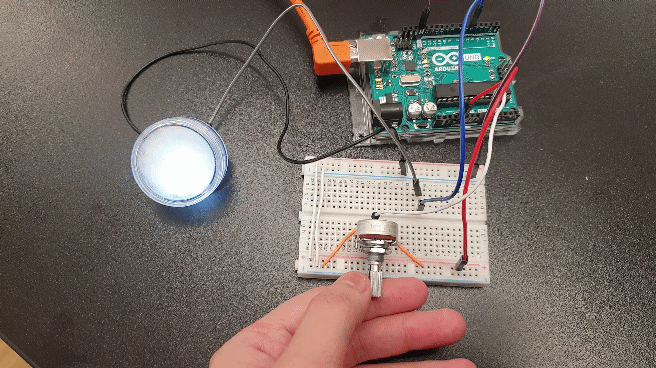top of page
Search

Prompt: Create a fading LED attached to a microcontroller. Your LED should be interruptible by either a pushbutton, analog input, or serial input.Modified code:

//xSquareFade code original by Tom Igoe 2019

//modified January 2020 by Nok

//set up pot for potentiometer and v for mapped value of potentiometer

int pot;

int v;

int currentLevel = 1;

int change = 1;

byte levelTable; // pre-calculated PWM levels

void setup() {

// put your setup code here, to run once:

// pre-calculated the PWM levels from the formula:

fillLevelTable();

}

void loop() {

// put your main code here, to run repeatedly:

//assign pot to pin A0 and map pot to v

v = map(pot, 0, 1023, 1, 10);

// decrease or increase by 1 point each time

// if at the bottom or top, change the direction:

if (currentLevel <= 0 || currentLevel >= 255) {

change = -change;

}

currentLevel += change;

//PWM output the result:

analogWrite(5, levelTable[currentLevel]*v);

delay(10);

Serial.println(levelTable[currentLevel]);

}

void fillLevelTable() {

//set the range of values:

float maxValue = 255;

//iterate over the array and calculate the right value for it:

for (int l = 0; l <= maxValue; l++) {

//square the current value:

float lightLevel = pow(l,2);

//map the result back to a 0-255 range:

lightLevel = map(lightLevel, 0, 65535, 0, 255);

levelTable[l] = lightLevel;

}

}

See All
Post: Blog2_Post
bottom of page